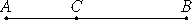# Proposition 76

If from a straight line there is subtracted a straight line which is incommensurable in square with the whole and which with the whole makes the sum of the squares on them added together rational, but the rectangle contained by them medial, then the remainder is irrational; let it be called minor.
X.33

From the straight line AB let there be subtracted the straight line BC which is incommensurable in square with the whole and fulfills the given conditions.

I say that the remainder AC is the irrational straight line called minor.Since the sum of the squares on AB and BC is rational, while twice the rectangle AB by BC is medial, therefore the sum of the squares on AB and BC is incommensurable with twice the rectangle AB by BC, and, in conversion, the sum of the squares on AB and BC is incommensurable with the remainder, the square on AC.

But the sum of the squares on AB and BC is rational, therefore the square on AC is irrational. Therefore AC is irrational.

Let it be called minor.

Q.E.D.

## Guide

This proposition is used for a few later propositions in Book X starting with X.82.